Mathematics Commons™

17,863 Full-Text Articles 17,120 Authors 4,137,002 Downloads285 Institutions

All Articles in Mathematics

17,863 full-text articles. Page 471 of 528.

2011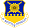Air Force Institute of Technology

Comparison Of Development Test And Evaluation And Overall Program Estimate At Completion, William R. Rosado

Theses and Dissertations

Historically, cost growth regression models analyze aggregate, program-level information. Initiatives by the Office of Secretary of Defense, Cost Assessment and Program Evaluation (OSD CAPE) require direct, centralized reporting of the complete Work Breakdown Structure (WBS) Earned Value (EV) data. Centralized reporting allows access to unfiltered, unaltered, EV data for multiple programs. Using regression, we evaluate if WBS element Development Test and Evaluation (DT&E) EV data is related to program estimate at completion (EAC). Identifying a relationship provides evidence validating pertinence and reliability of low level EV data. Additionally, a relationship between a specific WBS element and program EAC establishes ...

Operation Comics On Wkyu-Fm Public Radio, 2011Western Kentucky University

Operation Comics On Wkyu-Fm Public Radio, Dan Modlin, Bruce Kessler

Bruce Kessler

Operation Comics and their recent offering as NOOKbooks from the Barnes and Noble webpage were the subject of this interview for WKU's public radio, conducted by Dan Modlin.

Voter Model Perturbations And Reaction Diffusion Equations, 2011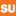Syracuse University

Voter Model Perturbations And Reaction Diffusion Equations, J. Theodore Cox, Richard Durrett, Edwin A. Perkins

Mathematics - Faculty Scholarship

We consider particle systems that are perturbations of the voter model and show that when space and time are rescaled the system converges to a solution of a reaction diffusion equation in dimensions d > 3. Combining this result with properties of the PDE, some methods arising from a low density super-Brownian limit theorem, and a block construction, we give general, and often asymptotically sharp, conditions for the existence of non-trivial stationary distributions, and for extinction of one type. As applications, we describe the phase diagrams of three systems when the parameters are close to the voter model: (i) a stochastic ...

Quantitative Interpretation Of A Genetic Model Of Carcinogenesis Using Computer Simulations, 2011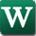Wright State University - Main Campus

Quantitative Interpretation Of A Genetic Model Of Carcinogenesis Using Computer Simulations, Donghai Dai, Brandon Beck, Xiaofang Wang, Cory Howk, Yi Li

Mathematics and Statistics Faculty Publications

The genetic model of tumorigenesis by Vogelstein et al. (V theory) and the molecular definition of cancer hallmarks by Hanahan and Weinberg (W theory) represent two of the most comprehensive and systemic understandings of cancer. Here, we develop a mathematical model that quantitatively interprets these seminal cancer theories, starting from a set of equations describing the short life cycle of an individual cell in uterine epithelium during tissue regeneration. The process of malignant transformation of an individual cell is followed and the tissue (or tumor) is described as a composite of individual cells in order to quantitatively account for intra-tumor ...

Wild Hypersurfaces, 2011Syracuse University

Wild Hypersurfaces, Andrew Crabbe, Graham J. Leuschke

Mathematics - Faculty Scholarship

Complete hypersurfaces of dimension at least 2 and multiplicity at least 4 have wild Cohen-Macaulay type.

2011Brigham Young University - Provo

Mean Square Estimate For Primitive Lattice Points In Convex Planar Domains, Ryan D. Coatney

Theses and Dissertations

The Gauss circle problem in classical number theory concerns the estimation of N(x) = { (m1;m2) in ZxZ : m1^2 + m2^2 <= x }, the number of integer lattice points inside a circle of radius sqrt(x). Gauss showed that P(x) = N(x)- pi * x satisfi es P(x) = O(sqrt(x)). Later Hardy and Landau independently proved that P(x) = Omega_(x1=4(log x)1=4). It is conjectured that inf{e in R : P(x) = O(x^e )}= 1/4. I. K atai showed that the integral from 0 to X of |P(x)|^2 dx = X^(3/2) + O(X(logX)^2). Similar results to those of the circle have been obtained for regions D in R^2 which contain the origin and whose boundary dD satis fies suff cient smoothness conditions. Denote by P_D(x) the similar error term to P(x) only for the domain D. W. G. Nowak showed that, under appropriate conditions on dD, P_D(x) = Omega_(x1=4(log x)1=4) and that the integral from 0 to X of |P_D(x)|^2 dx = O(X^(3/2)). A result similar to Nowak's mean square estimate is given in the case where only "primitive" lattice points, {(m1;m2) in Z^2 : gcd(m1;m2) = 1 }, are counted in a region D, on assumption of the Riemann Hypothesis.

A Primer On Chaos And Fractals, 2011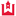Western Kentucky University

A Primer On Chaos And Fractals, Bruce Kessler

Mathematics Faculty Publications

This is a prelude to a performance of the play "Arcadia" exclusively for the science and math majors of Lipscomb University. One of the main characters of the story is a mathematical genius, and has realized the power and limitations of iterations in generating mathematical models and structures, although she is living in the early 1800's. This talk gives an introduction to the ideas of chaos theory, fractals, and randomness.

A Primer On Chaos And Fractals, 2011Western Kentucky University

A Primer On Chaos And Fractals, Bruce Kessler

Bruce Kessler

This is a prelude to a performance of the play "Arcadia" exclusively for the science and math majors of Lipscomb University. One of the main characters of the story is a mathematical genius, and has realized the power and limitations of iterations in generating mathematical models and structures, although she is living in the early 1800's. This talk gives an introduction to the ideas of chaos theory, fractals, and randomness.

Complete Characterizations Of Local Weak Sharp Minima With Applications To Semi-Infinite Optimization And Complementarity, 2011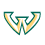Wayne State University

Complete Characterizations Of Local Weak Sharp Minima With Applications To Semi-Infinite Optimization And Complementarity, Boris S. Mordukhovich, Naihua Xiu, Jinchuan Zhou

Mathematics Research Reports

In this paper we identify a favorable class of nonsmooth functions for which local weak sharp minima can be completely characterized in terms of normal cones and subdifferentials, or tangent cones and subderivatives, or their mixture in finite-dimensional spaces. The results obtained not only significantly extend previous ones in the literature, but also allow us to provide new types of criteria for local weak sharpness. Applications of the developed theory are given to semi-infinite programming and to semi-infinite complementarity problems.

A Modification Of Extended Homoclinic Test Approach To Solve The (3+1)-Dimensional Potential-Ytsf Equation, 2011University of Kermanshah, Razi

A Modification Of Extended Homoclinic Test Approach To Solve The (3+1)-Dimensional Potential-Ytsf Equation, Mohammad Najafi, Mohammad Taghi Darvishi

By means of the extended homoclinic test approach (EHTA) one can solve some nonlinear partial differential equations (NLPDEs) in their bilinear forms. When an NLPDE has no bilinear closed form we can not use this method. We modify the idea of EHTA to obtain some analytic solutions for the (3+1)-dimensional potential-Yu- Toda-Sasa-Fukuyama (YTSF) equation by obtaining a bilinear closed form for it. By comparison of this method and other analytic methods, like HAM, HTA and three-wave methods, we can see that the new idea is very easy and straightforward

Benford’S Law Strikes Back: No Simple Explanation In Sight For Mathematical Gem, 2011University of Alberta

Benford’S Law Strikes Back: No Simple Explanation In Sight For Mathematical Gem, Arno Berger, Theodore P. Hill

Theodore P. Hill

No abstract provided.

The Schroedinger Equation With Potential In Rough Motion, 2011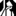University at Albany, State University of New York

The Schroedinger Equation With Potential In Rough Motion, Marius Beceanu, Avy Soffer

Mathematics and Statistics Faculty Scholarship

This paper proves endpoint Strichartz estimates for the linear Schroedinger equation in R 3 , with a time-dependent potential that keeps a constant profile and is subject to a rough motion, which need not be differentiable and may be large in norm. The potential is also subjected to a time-dependent rescaling, with a non-differentiable dilation parameter. We use the Strichartz estimates to prove the non-dispersion of bound states, when the path is small in norm, as well as boundedness of energy. We also include a sample nonlinear application of the linear results.

Nonsolvable Polynomials With Field Discriminant 5A, 2011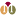University of Minnesota - Morris

Nonsolvable Polynomials With Field Discriminant 5A, David P. Roberts

Mathematics Publications

We present the first explicitly known polynomials in Z[x] with nonsolvable Galois group and field discriminant of the form ±pA for p ≤ 7 a prime. Our main polynomial has degree 25, Galois group of the form PSL2(5)5.10, and field discriminant 569. A closely related polynomial has degree 120, Galois group of the form SL2(5)5.20, and field discriminant 5311. We completely describe 5-adic behavior, finding in particular that the root discriminant of both splitting fields is 125 · 5-1/12500 ≈ 124.984 and the class number of the latter ...

Sharp Asymptotics Of The Lp Approximation Error For Interpolation On Block Partitions, 2011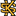Kennesaw State University

Sharp Asymptotics Of The Lp Approximation Error For Interpolation On Block Partitions, Yuliya Babenko, Tatyana Leskevich, Jean-Marie Mirebeau

Faculty Publications

Adaptive approximation (or interpolation) takes into account local variations in the behavior of the given function, adjusts the approximant depending on it, and hence yields the smaller error of approximation. The question of constructing optimal approximating spline for each function proved to be very hard. In fact, no polynomial time algorithm of adaptive spline approximation can be designed and no exact formula for the optimal error of approximation can be given. Therefore, the next natural question would be to study the asymptotic behavior of the error and construct asymptotically optimal sequences of partitions. In this paper we provide sharp asymptotic ...

Global Well-Posedness And Asymptotic Behavior Of A Class Of Initial-Boundary-Value Problems Of The Kdv Equation On A Finite Domain, 2011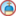University of Cincinnati - Main Campus

Global Well-Posedness And Asymptotic Behavior Of A Class Of Initial-Boundary-Value Problems Of The Kdv Equation On A Finite Domain, Ivonne Rivas, Muhammad Usman, Bingyu Zhang

Mathematics Faculty Publications

In this paper, we study a class of initial boundary value problem (IBVP) of the Korteweg- de Vries equation posed on a ?nite interval with nonhomogeneous boundary conditions. The IBVP is known to be locally well-posed, but its global L2 a priori estimate is not available and therefore it is not clear whether its solutions exist globally or blow up in finite time. It is shown in this paper that the solutions exist globally as long as their initial value and the associated boundary data are small, and moreover, those solutions decay exponentially if their boundary data decay exponentially.

Characterizing The Arc, 2011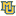Marquette University

Characterizing The Arc, Paul Bankston

Mathematics, Statistics and Computer Science Faculty Research and Publications

We offer a mathematical logic framework for talking about when a given topological space is characterizable relative to a“class of its peers.” The framework involves a relational alphabet for which there is a natural way of assigning relational structures to spaces in the peer class; and a putative characterization of the given space consists of a set of first-order sentences over that alphabet, all true for the space. The attempted characterization is a success if any peer space satisfying all the sentences is inevitably homeomorphic to the given space.

For example, it has long been known that the arc ...

2011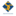Smith College

The Geometric And Dynamic Essence Of Phyllotaxis, Pau Atela

Mathematics and Statistics: Faculty Publications

We present a dynamic geometric model of phyllotaxis based on two postulates, primordia formation and meristem expansion. We find that Fibonacci, Lucas, bijugate and multijugate are all variations of the same unifying phenomenon and that the difference lies on small changes in the position of initial primordia. We explore the set of all initial positions and color-code its points depending on the phyllotactic type of the pattern that arises.

Signal And Noise In Complex-Valued Sense Mr Image Reconstruction, 2011Marquette University

Signal And Noise In Complex-Valued Sense Mr Image Reconstruction, Daniel B. Rowe, Iain P. Bruce

Mathematics, Statistics and Computer Science Faculty Research and Publications

In fMRI, brain images are not measured instantaneously and a volume of images can take two seconds to acquire at a low 64x64 resolution. Significant effort has been put forth on many fronts to decrease image acquisition time including parallel imaging. In parallel imaging, sub-sampled spatial frequency points are measured in parallel and combined to form a single image. Measurement time is decreased at the expense of increased image reconstruction difficulty and time. One significant parallel imaging technique known as SENSE utilizes a complex-valued regression coefficient estimation process with transposes replaced by conjugate transposes. However, in SENSE the noise structure ...

Common Hypercyclic Vectors For The Conjugate Class Of A Hypercyclic Operator, 2011Bowling Green State University - Main Campus

Common Hypercyclic Vectors For The Conjugate Class Of A Hypercyclic Operator, Kit C. Chan, Rebecca Sanders

Mathematics, Statistics and Computer Science Faculty Research and Publications

Given a separable, infinite dimensional Hilbert space, it was recently shown by the authors that there is a path of chaotic operators, which is dense in the operator algebra with the strong operator topology, and along which every operator has the exact same dense Gδ set of hypercyclic vectors. In the present work, we show that the conjugate set of any hypercyclic operator on a separable, infinite dimensional Banach space always contains a path of operators which is dense with the strong operator topology, and yet the set of common hypercyclic vectors for the entire path is a dense ...

Semidistributive Inverse Semigroups, Ii, 2011Marquette University

Semidistributive Inverse Semigroups, Ii, Kyeong Hee Cheong, Peter Jones

Mathematics, Statistics and Computer Science Faculty Research and Publications

The description by Johnston-Thom and the second author of the inverse semigroups S for which the lattice LJ(S) of full inverse subsemigroups of S is join semidistributive is used to describe those for which (a) the lattice L(S) of all inverse subsemigroups or (b) the lattice lo(S) of convex inverse subsemigroups have that property. In contrast with the methods used by the authors to investigate lower semimodularity, the methods are based on decompositions via GS, the union of the subgroups of the semigroup (which is necessarily cryptic).